# 關於視覺化與機器學習¶

:

from IPython.display import YouTubeVideo
import numpy as np
import requests
import pandas as pd
import matplotlib.pyplot as plt
from sklearn.linear_model import LinearRegression
from sklearn.linear_model import LogisticRegression


## 一個資料科學專案¶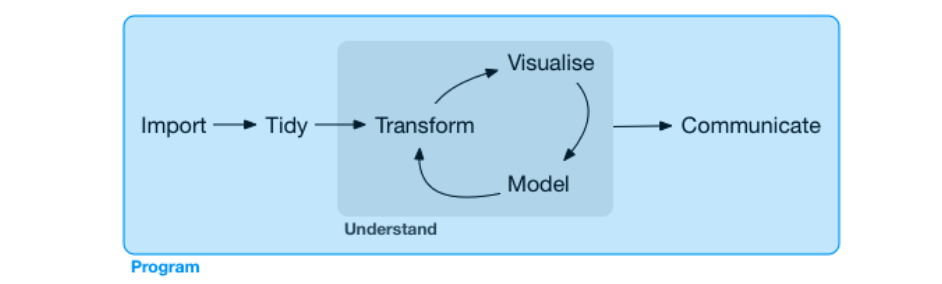## 何謂視覺化¶

I know having the data is not enough, I have to show it in a way that people both enjoy and understand.

Hans Rosling

1. 拿破崙征俄戰爭：法國土木工程師 Charles Minard 使用一種前所未見的帶狀圖來描繪拿破崙的軍隊從波蘭前進至俄羅斯邊界在特定地理位置的軍隊規模，在一個圖形上涵蓋七個資料特徵：軍隊人數、行軍距離、溫度、經度、緯度、行進方向以及特定日期，讀者可以一目瞭然 1812 年征俄戰爭中拿破崙軍隊的慘烈戰況。這樣外觀的帶狀圖在後來被稱為 Sankey 圖，以發明者 Matthew Henry Phineas Riall Sankey 作為命名，特別用來描述數量的流動與多寡。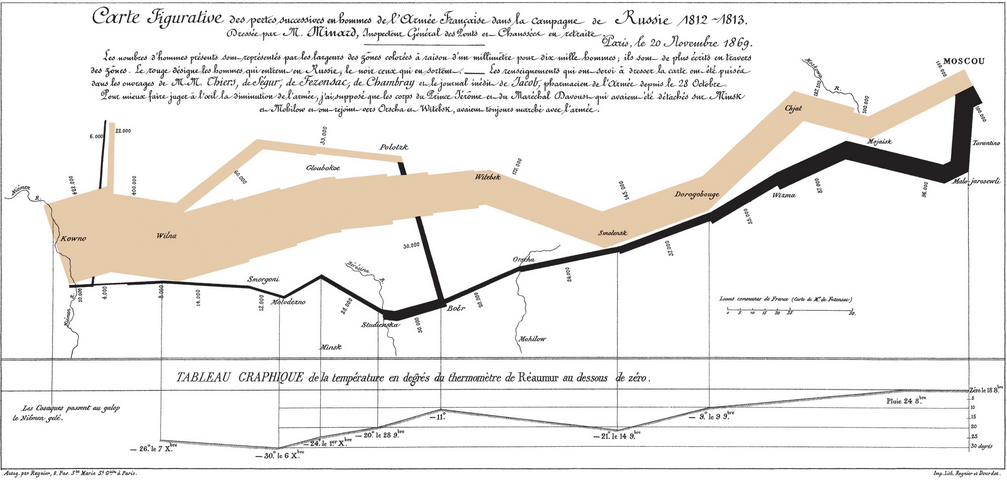1. 兩百年四分鐘：瑞典公衛教授 Hans Rosling 使用氣泡圖搭配動畫僅花費四分鐘和觀眾說明全世界超過兩百個國家在近兩百年中財富與健康程度的消長趨勢，在一個圖形上涵蓋五個資料特徵：人均國內生產總值、人均預期壽命、人口數、洲別、年份，觀眾可以一目暸然在綠能、和平、貿易與科技的助瀾下，長期世界國家的發展趨勢是往富裕且健康的方向前進。

:

YouTubeVideo('Z8t4k0Q8e8Y', width=640, height=360)

:


## 為何視覺化¶

### 原始資料¶

:

arr = np.random.normal(size=10000)
arr

:

array([ 0.28763377, -0.12276065,  0.34811166, ...,  1.85008303,
-0.05646011,  0.94117921])


:

fig = plt.figure()
ax = plt.axes()
ax.hist(arr, bins=50)
plt.show()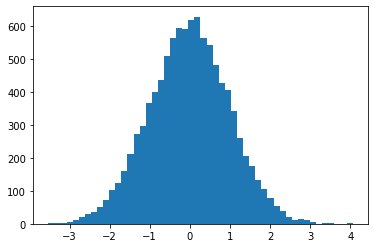### 函式¶

:

eps = 1e-06 # epsilon, 一個很微小的數字避免 0 輸入 log 函式後產生無限大
p = np.linspace(0 + eps, 1 - eps, 10000)
log_loss_0 = -np.log(1-p)
log_loss_1 = -np.log(p)
print(p)
print(log_loss_0)
print(log_loss_1)

[1.00000000e-06 1.01009801e-04 2.01019602e-04 ... 9.99798980e-01
9.99898990e-01 9.99999000e-01]
[1.00000050e-06 1.01014903e-04 2.01039809e-04 ... 8.51210813e+00
9.20029301e+00 1.38155106e+01]
[1.38155106e+01 9.20029301e+00 8.51210813e+00 ... 2.01039809e-04
1.01014903e-04 1.00000050e-06]


:

fig = plt.figure()
ax = plt.axes()
ax.plot(p, log_loss_0, label='$y_{true}=0$')
ax.plot(p, log_loss_1, label='$y_{true}=1$')
ax.legend()
plt.show()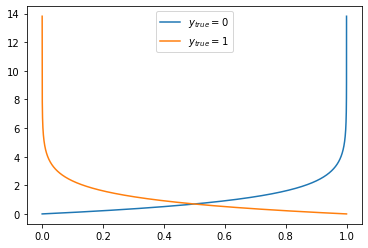### 數學式¶

\begin{equation} f(x) = \frac{1}{1+e^{-x}} \end{equation}

:

x = np.linspace(-6, 6, 1000)
fx = 1 / (1 + np.exp(-x))
fig = plt.figure()
ax = plt.axes()
ax.plot(x, fx)
plt.show()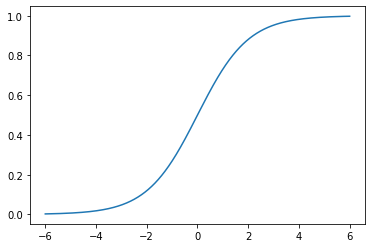## 何謂機器學習¶

A computer program is said to learn from experience E with respect to some class of tasks T and performance measure P if its performance at tasks in T, as measured by P, improves with experience E.

Tom Mitchel

\begin{equation} y = f(X) \end{equation}

\begin{equation} \hat{y} = h(X; w) \end{equation}

\begin{equation} H = \{h_1(X; w), h_2(X; w), ..., h_n(X; w)\} \end{equation}

\begin{equation} Minimize \; \frac{1}{m}\sum_{i}{(y^{(train)}_i - \hat{y_i}^{(train)})^2} \end{equation}

\begin{equation} Minimize \; \sum_{i} \mid y^{(train)}_i \neq \hat{y_i}^{(train)} \mid \end{equation}

• 監督式學習：訓練資料中具備已實現的數值或標籤

• 迴歸：數值預測的任務

• 分類：類別預測的任務

• 非監督式學習：訓練資料中「不」具備已實現的數值或標籤

## pyvizml 模組¶

from pyvizml import CreateNBAData


:

class CreateNBAData:
"""
This class scrapes NBA.com offical api: data.nba.net.
See https://data.nba.net/10s/prod/v1/today.json
Args:
season_year (int): Use the first year to specify season, e.g. specify 2019 for the 2019-2020 season.
"""
def __init__(self, season_year):
self._season_year = str(season_year)

def create_players_df(self):
"""
This function returns the DataFrame of player information.
"""
request_url = "https://data.nba.net/prod/v1/{}/players.json".format(self._season_year)
resp_dict = requests.get(request_url).json()
players_list = resp_dict['league']['standard']
players_list_dict = []
print("Creating players df...")
for p in players_list:
player_dict = {}
for k, v in p.items():
if isinstance(v, str) or isinstance(v, bool):
player_dict[k] = v
players_list_dict.append(player_dict)
df = pd.DataFrame(players_list_dict)
filtered_df = df[(df['isActive']) & (df['heightMeters'] != '')]
filtered_df = filtered_df.reset_index(drop=True)
self._person_ids = filtered_df['personId'].values
return filtered_df

def create_stats_df(self):
"""
This function returns the DataFrame of player career statistics.
"""
self.create_players_df()
career_summaries = []
print("Creating player stats df...")
for pid in self._person_ids:
request_url = "https://data.nba.net/prod/v1/{}/players/{}_profile.json".format(self._season_year, pid)
response = requests.get(request_url)
profile_json = response.json()
career_summary = profile_json['league']['standard']['stats']['careerSummary']
career_summaries.append(career_summary)
stats_df = pd.DataFrame(career_summaries)
stats_df.insert(0, 'personId', self._person_ids)
return stats_df

def create_player_stats_df(self):
"""
This function returns the DataFrame merged from players_df and stats_df.
"""
players = self.create_players_df()
stats = self.create_stats_df()
player_stats = pd.merge(players, stats, left_on='personId', right_on='personId')
return player_stats


CreateNBAData 需要傳入參數球季年份進行初始化，舉例我們要擷取的若是 2019-2020 球季，初始化類別就輸入 2019。這個類別定義了三個方法，create_players_df() 會回傳球員資料框、create_stats_df() 會回傳球員生涯攻守統計資料框、create_player_stats_df() 則會將球員資料框與球員生涯攻守統計資料框內部聯結（Inner join）後回傳。其中 create_stats_df()create_player_stats_df() 兩個方法因為要對 data.nba.net 發出數百次的 HTTP 請求，等待時間會較長，要請讀者耐心等候。

:

cnd = CreateNBAData(2019)
player_stats = cnd.create_player_stats_df()

Creating players df...
Creating players df...
Creating player stats df...


## 為何機器學習¶

### 判斷質數¶

:

def f(x):
"""
判斷輸入 x 是否為質數，是質數則輸出 1，否則輸出 0
"""
n_divisors = 0
for i in range(1, x+1):
if x % i == 0:
n_divisors += 1
if n_divisors > 2:
break
return int(n_divisors == 2)
print(bool(f(1))) # 非質數
print(bool(f(2))) # 質數
print(bool(f(3))) # 質數

False
True
True


### 數值預測：球員的體重為何？¶

:

X = player_stats['heightMeters'].values.reshape(-1, 1)
y = player_stats['weightKilograms'].values
lr = LinearRegression()
h = lr.fit(X, y)
print(h.predict(np.array([[1.90]]))) # 預測身高 190 公分 NBA 球員的體重
print(h.predict(np.array([[1.98]]))) # 預測身高 198 公分 NBA 球員的體重
print(h.predict(np.array([[2.03]]))) # 預測身高 203 公分 NBA 球員的體重

89.89882199692715
97.66998879805146
102.52696804875413


### 類別預測：球員的鋒衛位置為何？¶

:

unique_pos = player_stats['pos'].unique()
pos_dict = {i: p for i, p in enumerate(unique_pos)}
pos_dict_reversed = {v: k for k, v in pos_dict.items()}
print(pos_dict)
print(pos_dict_reversed)

{0: 'G', 1: 'C', 2: 'C-F', 3: 'F-C', 4: 'F', 5: 'F-G', 6: 'G-F'}
{'G': 0, 'C': 1, 'C-F': 2, 'F-C': 3, 'F': 4, 'F-G': 5, 'G-F': 6}

:

X = player_stats[['apg', 'rpg']].values
pos = player_stats['pos'].map(pos_dict_reversed)
y = pos.values
logit = LogisticRegression()
h = logit.fit(X, y)
print(pos_dict[h.predict(np.array([[5, 5]]))]) # 預測場均助攻 5 場均籃板 5 的 NBA 球員鋒衛位置
print(pos_dict[h.predict(np.array([[5, 10]]))]) # 預測場均助攻 5 場均籃板 10 的 NBA 球員鋒衛位置
print(pos_dict[h.predict(np.array([[5, 15]]))]) # 預測場均助攻 5 場均籃板 15 的 NBA 球員鋒衛位置

G
F
C


## 延伸閱讀¶

2. Hans Rosling (https://en.wikipedia.org/wiki/Hans_Rosling)

3. Charles Minard (https://en.wikipedia.org/wiki/Charles_Joseph_Minard)

4. Matthew Henry Phineas Riall Sankey (https://en.wikipedia.org/wiki/Matthew_Henry_Phineas_Riall_Sankey)

5. Hans Rosling: 200 years in 4 minutes - BBC News (https://www.youtube.com/watch?v=Z8t4k0Q8e8Y)

6. Tom M. Mitchell (https://en.wikipedia.org/wiki/Tom_M._Mitchell)

7. Normal distribution (https://en.wikipedia.org/wiki/Normal_distribution)

8. data.nba.net (https://data.nba.net/10s/prod/v1/today.json)